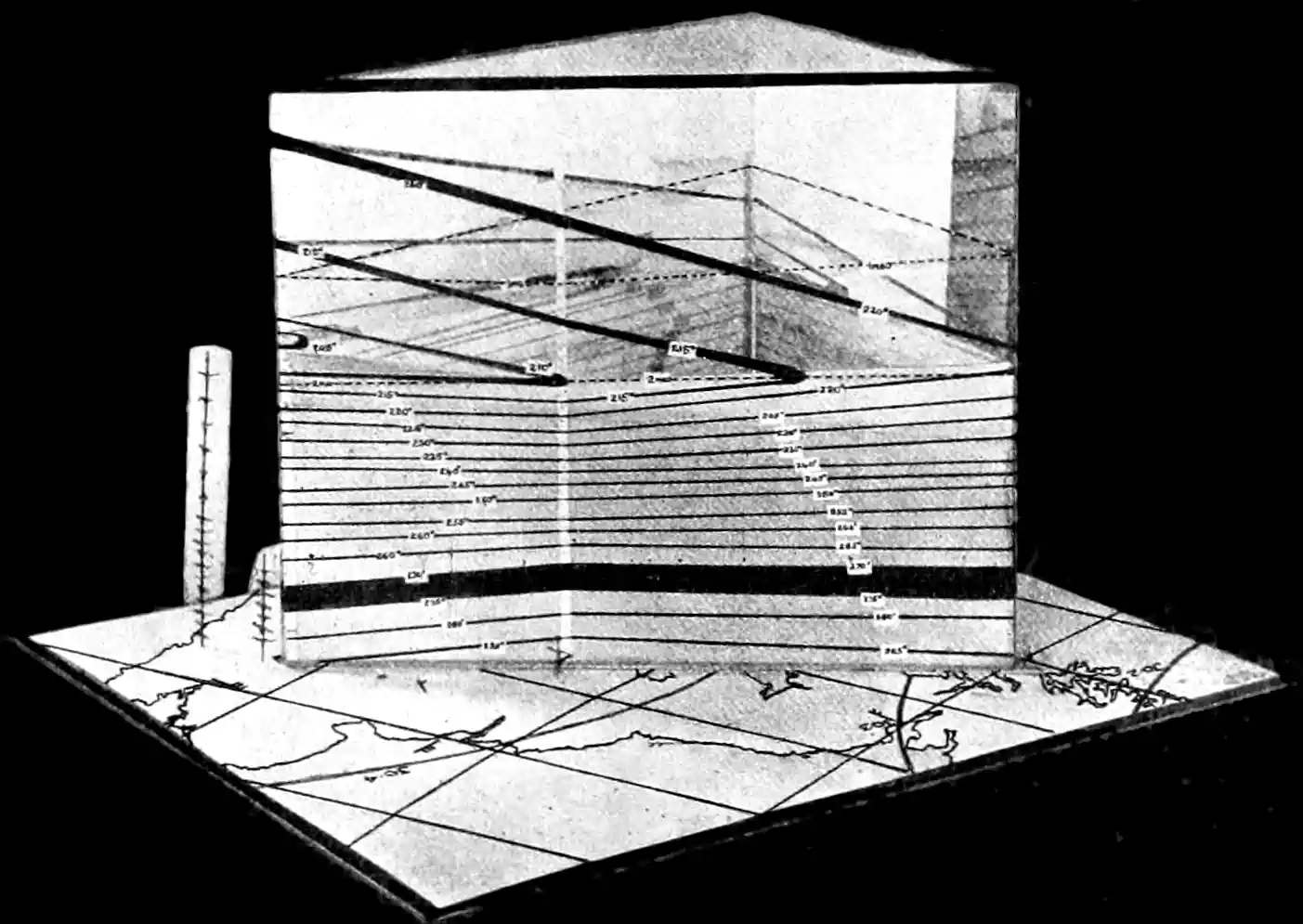Inverse problems for PDEs and other spatial models

a.k.a. Bayesian calibration, model uncertaintyInverse problems where the model is full of spatioemtporal correlations. I am particularly thinking about this in the context of PDE solvers, particularly approximate ones.

Suppose I have a PDE, possibly with some unknown parameters in the driving equation. All being equal I can do not too badly at approximating that with tools already mentioned. What if I wish simultaneously infer some unknown inputs? Then we consider it as an inverse problem. This is not quite the same as the predictive problem that many of the methods consider. However, we are free to use simulation-based inference to solve, or MCMC methods to do so for any of the forward-operator-learning approaches. To train the model to solve target the inverse problem directly, we might consider GANs or variational inference. At this point we are more or less required to start thinking about this in a probabilistic network or we will miss essential uncertainty quantification.

We are also encouraged to think about this as an approximation problem. We are surprised to find anything like a clean closed-form solution for the posterior distribution of some parameter in a PDE. Worse, the hypothetical solution is probably not even particularly computationally convenient. Why would it be? This will be especially trick in Bayesian inversion.

As far as how we might proceed, Liu, Yeo, and Lu (2020) is one approach, generalizing the approach of F. Sigrist, Künsch, and Stahel (2015b), but for advection/diffusion equations specifically. Generic methods include Bao et al. (2020); Jo et al. (2019); Lu, Mao, and Meng (2019); Raissi, Perdikaris, and Karniadakis (2019); Tait and Damoulas (2020); Xu and Darve (2020); Yang, Zhang, and Karniadakis (2020); Zhang, Guo, and Karniadakis (2020); Zhang et al. (2019).

Gaussian process case

Alexanderian (2021) states a ‘well-known’ result, that the solution of a Bayesian linear inverse problem with Gaussian prior and noise models is a Gaussian posterior measure $$\mu_{\text {post }}^{y}=\mathcal{N}\left(m_{\text {MAP }}, \mathcal{C}_{\text {post }}\right)$$, where $\mathcal{C}_{\text {post }}=\left(\mathcal{F}^{*} \boldsymbol{\Gamma}_{\text {noise }}^{-1} \mathcal{F}+\mathcal{C}_{\text {pr }}^{-1}\right)^{-1} \quad \text { and } \quad m_{\text {MAP }}=\mathcal{C}_{\text {post }}\left(\mathcal{F}^{*} \boldsymbol{\Gamma}_{\text {noise }}^{-1} \boldsymbol{y}+\mathcal{C}_{\text {pr }}^{-1} m_{\text {MAP }}\right)$

No comments yet. Why not leave one?

GitHub-flavored Markdown & a sane subset of HTML is supported.